• 在做统计报表的时候，通常会用到一些复杂的统计功能，而不是简单的 count(*), sum(abc) 就可以的，这就用到了一些基于条件的统计功能。 带条件count记录数 参考方法: 统计sub_type='REFUND_FEE’ 的记录数: ...
在做统计报表的时候，通常会用到一些复杂的统计功能，而不是简单的 count(*), sum(abc) 就可以的，这就用到了一些基于条件的统计功能。
带条件取count记录数
参考方法:
统计sub_type='REFUND_FEE’ 的记录数:

方法一.select count(sub_type) from t where t.sub_type='REFUND_FEE’；
方法二.select sum(if( B.sub_type='REFUND_FEE’ ,1,0)) from t；
方法三.select count(B.sub_type=‘REFUND_FEE’ or null) from t；

解释：
当select B.sub_type=‘REFUND_FEE’ 时：
如果sub_type为REFUND_FEE则返回1，
不空且不为REFUND_FEE否则返回0，
空时返回null。
所以B.sub_type=‘REFUND_FEE’ or null 只返回sub_type=‘REFUND_FEE’ 的，其余的都返回null ,而count(列名)时是不会统计null的个数的
注：count(*)会把null的个数也统计在内

项目sql

SELECT subjectName,doctorName,count(1) AS sumNum,
count(OVERTIMES>0 or null)as overNum            //只统计OVERTIMES>0的数
from ht_personstream
WHERE 1=1

and subjectId = #{subjectId}

= #{startTime} ]]>        //mysql日期格式化
GROUP BY  doctorId,subjectId

项目sql百分数保留两位小数

SELECT
subjectName as name,
concat(convert(((SUM(OVERTIMES)/(count(1)+SUM(OVERTIMES)))*100 ),decimal(10,2)),'%') as VALUE
FROM HT_PERSONSTREAM
where isDeleted = 0
GROUP BY subjectId,doctorId

//利用在一条语句中count出不同的条件,记录一下 ..
SELECT
SUM( goods_amount ) AS money,
count( * ) AS num,
count(if(pay_status=1,true,null)) AS success,
count(if(pay_status=2,true,null)) AS fall
FROM tab_order_info
WHERE user_id = 11

SUM()函数按条件求和
一般求和

select sum(money) from user group by id;

按条件求和

select sum(if(type=1,money,0)) from user group by id;


展开全文mysql
• Hibernate（分组查询等续）  使用聚集函数 ...Sum：求和函数 Avg：求平均数函数  Count：统计函数 Session session = HibernateSessionFactory.getSession(); Transaction tx = ses


Hibernate（分组查询等续）
使用聚集函数

在HQL中可以调用 Count：统计函数 Min：求最小值函数 Max：求最大值函数 Sum：求和函数 Avg：求平均数函数
Count：统计函数 Session session = HibernateSessionFactory.getSession(); Transaction tx = session.beginTransaction(); Integer count = (Integer)session.createQuery("select count(*) from Hx") .uniqueResult(); System.out.print(count); tx.commit(); session.close(); Avg：求平均数函数 Session session = HibernateSessionFactory.getSession(); Transaction tx = session.beginTransaction(); Float count = (Float)session.createQuery("select avg(c.id) from Hx c")  .uniqueResult(); System.out.print(count); tx.commit(); session.close(); Sum：求和函数 Session session = HibernateSessionFactory.getSession(); Transaction tx = session.beginTransaction(); Integer count = (Integer)session.createQuery("select sum(c.id) from Hx c")  .uniqueResult(); System.out.print(count); tx.commit(); session.close(); Min：求最小值函数 Max：求最大值函数 Session session = HibernateSessionFactory.getSession(); Transaction tx = session.beginTransaction(); Object[] count = (Object[])session.createQuery("select min(c.age),max(c.age) from Hx c") .uniqueResult(); String min = (String)count; String max = (String)count; System.out.print("min="+min+"|max="+max); tx.commit(); session.close(); 分组查询 Session session = HibernateSessionFactory.getSession(); Transaction tx = session.beginTransaction(); Iterator it = session.createQuery("select c.name,count(c) from Hx c group by c.name") .iterate(); while(it.hasNext()) { Object[] oc = (Object[])it.next(); String count = (Integer)oc;  System.out.println(name+":"+count); } tx.commit(); session.close();


展开全文• 背景：mysql 查询语句中where条件相同，sum、count函数中需要使用有个不同条件。test表内容：目标：查询class=1、mon&gt;=2并且score&gt;=70的score总和、被求和的score个数if 语句实现：case when 语句...
背景：mysql 查询语句中where条件相同，sum、count函数中需要使用有多个不同条件。test表内容：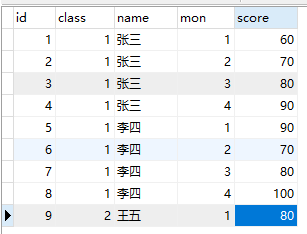目标：查询class=1、mon>=2并且score>=70的score总和、被求和的score个数if 语句实现：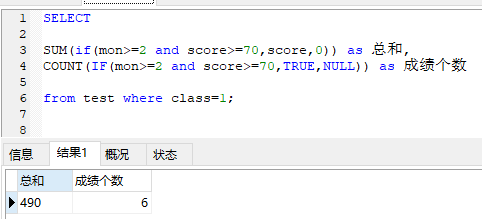说明：

if(mon>=2 and score>=70,score,0) ，当 mon>=2 and score>=70 两个条件同时成立，sum计算score值，否则计算0；IF(mon>=2 and score>=70,TRUE,NULL)，当 mon>=2 and score>=70 两个条件同时成立，count统计，否则不统计。
case when 语句实现：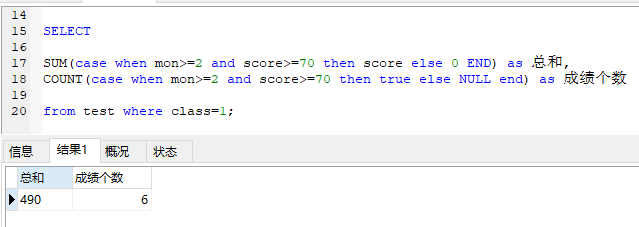说明：同上。
展开全文mysql if case when
• 首先在写这博客的初衷是因为在工作开发过程中遇到了数据汇总问题，并且有的还是按条件汇总，这就有点小麻烦，然而学会使用sum函数之后，这些问题就迎刃而解了。是不是很Nice。好记性不如烂笔头！再此，希望能帮到...
首先在写这博客的初衷是因为在工作开发过程中遇到了数据汇总问题，并且有的还是按条件汇总，这就有点小麻烦，然而学会使用sum函数之后，这些问题就迎刃而解了。是不是很Nice。好记性不如烂笔头！再此，希望能帮到类似需求的小伙伴，谢谢！！！下面进入正题。
一、汇总数据展示：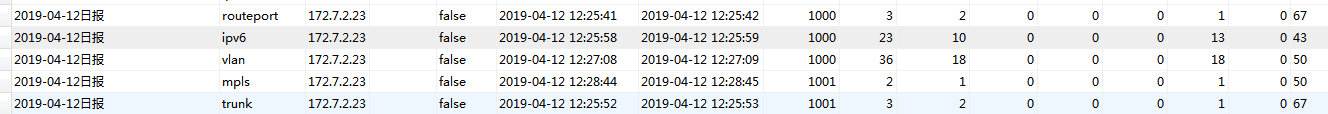二、后台数据封装类：
public class FtDailyStaticData {

private int moduleTotal;//模块总数
private int modulePass;//模块通过的数量
private int caseTocal;//用例总数
private int casePassed;//用例通过数
private int lineCover;//代码通过行数
private int lineTotal;//代码总行数
private int funcCover;//函数通过数
private int funcTocal;//函数总数
}
//set、get方法省略
三、sql语句编写：
<select id="findSum" parameterType="java.lang.Integer" resultType="java.util.Map">
SELECT COUNT(1) AS moduleTocal,
SUM(IF(result='true',1,0)) AS modulePass,
<!--SUM(CASE WHEN result = 'true' THEN 1 ELSE 0 END) AS passtotal,-->
SUM(total) AS caseTocal,
SUM(passed) AS casePass,
SUM(line_total) ,
SUM(line_cover),
SUM(func_cover),
SUM(func_total)
FROM ft_build_info WHERE build_id  = #{buildId}
</select>
其中第三行和第四行的代码作用一样，只是两种不同的函数。这就是按条件汇总数据的函数：
SUM(IF(result='true',1,0)) AS modulePass,         SUM(CASE WHEN result = 'true' THEN 1 ELSE 0 END) AS passtotal,
注意：sum（）是一个函数，在取值的时候要用map取值。这两个函数挺重要的，建议理解掌握
四、数据访问层
public interface FtBuildInfoDao {

//这里用arrayList接受，是用来保证查询的数据是升序还是降序处理的
//ArrayList<Map<String,Object>> findSum(Integer buildId);

Map<String,Object> findSum(Integer buildId);
}

注意：用ArrayList接受Map做泛型的作用，如果没有要求对数据进行排序的要求，直接可以选择用Map进行接收！
五、业务层（取值）：
public FtDailyStaticData findSum(Integer buildId) {
//ArrayList<FtDailyStaticData> lists = new ArrayList<FtDailyStaticData>();
//ArrayList<Map<String, Object>> list = ftTestDao.findSum(buildId);
Map<String, Object> map = ftTestDao.findSum(buildId);
FtDailyStaticData ftDailyStaticData = new FtDailyStaticData();
//for (Map<String, Object> map : list) {
Object object = map.get("moduleTocal");
int moduleTocal = Integer.parseInt(object.toString());
ftDailyStaticData.setModuleTotal(moduleTocal);//统计模块总数

ftDailyStaticData.setModulePass(Integer.parseInt(map.get("modulePass").toString()));
ftDailyStaticData.setCaseTocal(Integer.parseInt(map.get("caseTocal").toString()));
ftDailyStaticData.setCasePassed(Integer.parseInt(map.get("casePass").toString()));
ftDailyStaticData.setLineTotal(Integer.parseInt(map.get("SUM(line_total)").toString()));
ftDailyStaticData.setLineCover(Integer.parseInt(map.get("SUM(line_cover)").toString()));
ftDailyStaticData.setFuncTocal(Integer.parseInt(map.get("SUM(func_total)").toString()));
ftDailyStaticData.setFuncCover(Integer.parseInt(map.get("SUM(func_cover)").toString()));
//}
return ftDailyStaticData;
}
}
注意 ：map取值的时候根据你sql查询的结果字段名进行取值，否则取不到相关的信息！！
六、控制层省略，直接调用业务层方法即可
七、测试：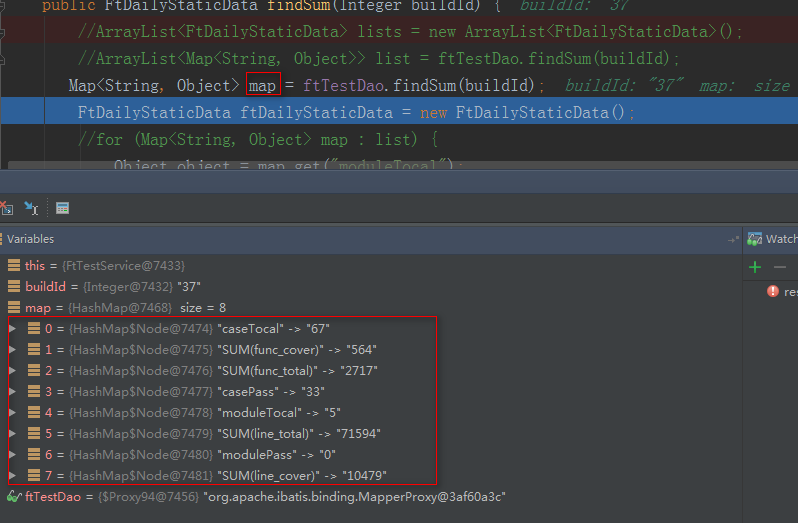成功返回，nb。
引申：

SELECT
t.*,
CONCAT (ROUND (IFNULL((t.Pass * 100 / t.Total), 0),2),'%') As Rate <!--求百分比并且保留两位小数-->
from
(
SELECT
COALESCE( SUM(total),0 ) As Total,  <!--这个意思是当total为null时返回0-->
COALESCE( SUM(passed),0 ) AS Pass,
COALESCE( SUM(error),0 ) AS Error
FROM ft_build_info fb
<where>
<if test="project != null and project.size !=0">
and fb.ft_project_name in
<foreach collection="project" item="item" open="(" separator="," close=")">
#{item}
</foreach>
</if>
<if test="version != null and version.size !=0">
and fb.ft_version in
<foreach collection="version" item="item" open="(" separator="," close=")">
#{item}
</foreach>
</if>
<if test="moduleName != null and moduleName.size !=0">
and fb.module_name in
<foreach collection="moduleName" item="item" open="(" separator="," close=")">
#{item}
</foreach>
</if>
<if test="type != null and type.length !=0">
and fb.flag in
<foreach collection="type" item="item" open="(" separator="," close=")">
#{item}
</foreach>
</if>
<if test="beginTime != null and beginTime != ''">
and <![CDATA[fb.begin_time >= #{beginTime}]]>
</if>
<if test="endTime != null and endTime != ''">
and <![CDATA[fb.end_time <= #{endTime}]]>
</if>
</where>
) t

字符串日期格式的比较大小：
SELECT  *  FROM  ft_build_info fb  WHERE fb.begin_time >= CONCAT(DATE_FORMAT(DATE_ADD(NOW(),INTERVAL 0 DAY),'%Y-%m-%d'), ' 00:00:00')  AND fb.end_time <= CONCAT(DATE_FORMAT(DATE_ADD(NOW(),INTERVAL 0 DAY),'%Y-%m-%d'),' 23:59:59')
DATE_SUB（）函数是在该日期上减去一个时间数，INTERVAL 后为负数就是加，为正数是减。

在做求和并且计算百分比的时候出现了一个问题，记录一下！！
修改之前的sql：

SELECT t.* , CONCAT (ROUND (IFNULL((t.LineCover * 100 / t.LineTotal), 0.00),2),'%' ) As LineRate FROM ( SELECT COALESCE( SUM(fb.line_total),0 ) As LineTotal,  COALESCE( SUM(fb.line_cover),0 ) AS LineCover, CONCAT(fb.module_name,'') AS moduleName FROM  ft_build_info fb WHERE  fb.flag = 1 GROUP BY fb.module_name  ) t WHERE  1=1 ORDER BY LineRate desc LIMIT 10

结果如下：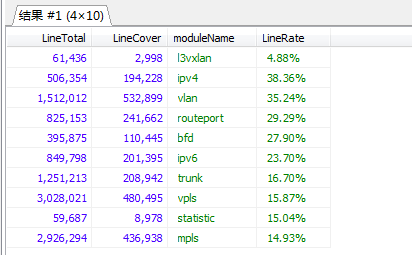很奇怪，我明明是按照LineRate降序排列 的，为什么第一条数据排在第一条，很明显不对的。这是因为我们在使用concat函数时拼接了一个‘%’，这样在后续使用ORDER BY LineRate的时候，就会以字符串形式进行排序，怎么解决呢？
方法如下 ：
方法一：ORDER BY '123'+0;（首推）
方法二：ORDER BY '123'*1;
方法三：ORDER BY CAST('123' AS SIGNED); 方法四：ORDER BY CONVERT('123',SIGNED);
注意：其中'123'是你要排序的字段。
演示第一种方法：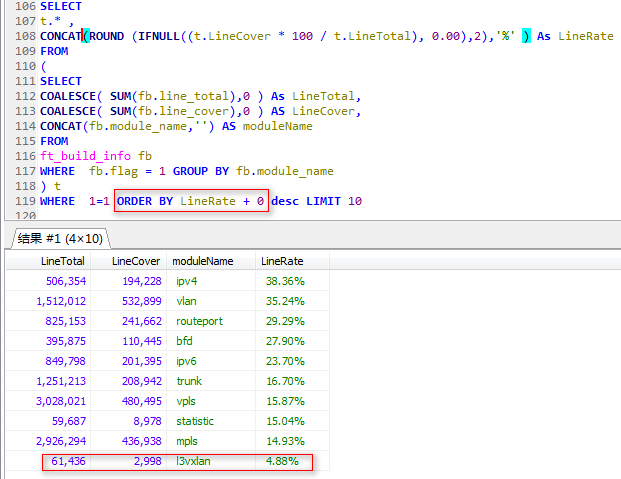展开全文• 聚合函数中如果想汇总某一类数据，可以在括号中增加条件： sum(casewhen字段>0then1else0end)as字段 ...count函数不管记录内容是0或1，它的作用只是计算记录数，如果你要计算次数，用sum(casewhen字段>0then1...
• ## sql 多条件求和

千次阅读 2019-06-03 17:14:52
,sum(case when type in (1,2) then [count] else 0 end) as sum1 ,sum(case when type in (3) then [count] else 0 end) as sum2 ,sum(case when type in (4,5) then [count] else 0 end) as sum3 from 表名 ...sql
• 首先举个栗子（不想看的话直接下面看总结）： order_type：订单类型 open_id：用户唯一标识 SELECT  date(create_time) AS '当天...sum函数中使用if判断条件：{ sum( IF (order_type = 0, real_price, 0) ) A...MySQL java 数据库 sum函数
• 统计个数count函数: #统计表总行数: select count(*) from user; select count(id) from user; #统计符合条件的行数: select count(*) from user where id>2; 求和sum(): select sum(id) from user; 平均值avg():...数据库
• 条件求和 (对所有金额大于100的数据进行求和) select sum(if(money>=100,money,0)) from user group by id; 聚合函数中如果想汇总某一类数据的数量，可以在括号中增加条件： sum(case when 字段>0 then 1 ...mysql
• ## 利用Stream聚合函数对BigDecimal求和

万次阅读 多人点赞 2018-02-26 11:33:52
数据库查找的结果经常会有List等集合，而集合中存放法是JAVA对象，对象中存在BigDecimal的字段，如果用for或者iterator遍历来累加感觉很麻烦，stream聚合函数很好的解决了这个问题．做个笔记mark一下 POJO ...
• 这时候就要用到多条件的判定选择、求和、计数等IFS函数。【例1】多条件判定(IFS函数)，根据销售额和提成比例，求算销售提成。思考：判定销售额位于的区间，并运算返回相应的销售提成。也就是小于5000，提成12%，小于...
• 最近在使用JPQL时遇到了一个问题，关于count函数和sum函数不知该如何取值，在网上查了查资料，找到了一种解决方法   具体代码如下：     public long ...{  //queryString = ...jpa null string list
• create_time 为数据库一时间相关字段,需要根据这个字段将查询到的数据按照年(月/日... SELECT DATE_FORMAT(create_time,'%Y%m%d') days,COUNT(id) COUNT FROM role GROUP BY days; SELECT DATE_FORMAT(create_time,...mysql使用
• oracle数据库对test_table表的三个列count1，count2，count3求sum的两种sql，做个记录 第一种 select sum (case when count1 is not null then count1 when count2 is not null then count2 when count3 is not ...统计报表
• if (m.count(x)) return m[x]; for (i = 2;j < x;i = j + 1) { j = x / (x / i); ans -= mobius(x / i) * (j - i + 1); } m[x]=ans; return ans; } int main() { long long i,j,a,b; scanf("%I64d%I64...shell
• SELECT COUNT(CASE WHEN category LIKE '%外购附件%' THEN 1 END) wgfjcount, COUNT(CASE ...sql
• Private Sub Button2_Click(sender As Object, e As...xlSheets.Application.WorksheetFunction.Sum(xlSheet.Range("c1:c1000")) '利用sum函数 求和 xlBook.Close(True) '关闭工作簿 xlApp.Quit '结束EXCEL对象 End Sub
• 聚合函数 聚合函数对一组值进行计算并返回单一的值，通常聚合函数...SUM(求和)函数 SUM函数返回表达式中所有值的和或仅非重复值的和。SUM 只能用于数字列。空值将被忽略。 参数说明： ALL：对所有的值应用此聚合函数...sum max avg
• COUNT 函数COUNT(区域）COUNT 函数只能用于计算区域中包含数值的单元格的个数，不能用于统计文本型数据。COUNTA 函数COUNTA(区域）使用方式与 COUNT函数相...python 数据分析 算法 机器学习 excel
• sum()函数count()函数的区别： 1）求和用累加sum(),求行的个数用累计count() 2）数据库中对空值的处理：sum()不计算，count()认为没有此项； 示例： SUM是对符合条件的记录的数值列求和 COUNT 是对查询中符合...mysql
• #include <stdio.h> #include <stdlib.h> int fun4(int m,int a[]) { int i;... int count=0; for(i=1;i<=m;i++) { if(i%7==0||i%11==0){ a[i]=i; printf("%d\t",a[i]); ...
• 1、mysql 数据库中 count() 函数是统计查询结果中的行数，例如我们有下表 user_auth ： 使用 count() 函数来查询结果个数，使用如下查询： mysql &gt; select count(*) from user_auth ;     注意的...mysql sum
• 当我们需要在一条Sql语句中进行不同条件的统计时，单独的COUNT()无法满足我们的需求，这时可以通过SUM()来完成。SELECT CourseID, TotalCount=COUNT(*), UnreadCount=SUM(CASE WHEN ReadStatus=0 THEN 1 ELSE 0 ...SUM
• 技巧360 按颜色求和与计数函数技巧360 按颜色求和与计数函数
• count是sql的一个标准行统计函数，在实际应用中很是普遍，还有另外一种情况也经常会被用到：有条件地统计行数。除此之外，count统计时会跳过null值，部分count可用sum求和代替。mysql sum
• 在《【Python】利用setdefault函数实现dict的转置（key与value对互换）...本文介绍如何利用list自带的count函数，来统计list中每个元素出现的次数。 具体代码如下： arr_appear=dict((a,arr.count(a)) for a in arr);Python list
• using System; using System.Collections.Generic; using System.Linq; using System.Text; using System.Threading.Tasks; ...namespace 函数练习 ... //阶乘累加求和 没有返回值，没有参数 ...
• 主要介绍了Windows 10，MySQL版本是5.7.12-log环境下mysql中聚合函数count的使用和性能优化,需要的朋友可以参考下...